Processing ......FreeComputerBooks.com Links to Free Computer, Mathematics, Technical Books all over the World

Applied Nonparametric Regression
🌠 Top Free Computer Networking Books - 100% Free or Open Source!
• Title Applied Nonparametric Regression
• Author(s) Wolfgang Haerdle
• Publisher: Cambridge University Press (January 31, 1992), eBook (January 27, 2004 )
• Paperback 352 pages
• Language: English
• ISBN-10: 0521429501
• ISBN-13: 978-0521429504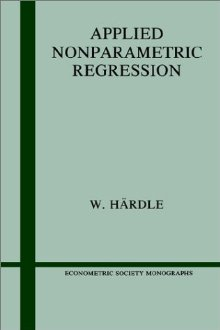Book Description

Applied Nonparametric Regression brings together in one place the techniques for regression curve smoothing involving more than one variable. The computer and the development of interactive graphics programs has made curve estimation popular. This volume focuses on the applications and practical problems of two central aspects of curve smoothing: the choice of smoothing parameters and the construction of confidence bounds.

The methods covered in this text have numerous applications in many areas using statistical analysis. Examples are drawn from economics - such as the estimation of Engel curves - as well as other disciplines including medicine and engineering. For practical applications of these methods a computing environment for exploratory Regression - XploRe - is described.

The material is easy to accomplish since the e-book character of the text gives a maximum of flexibility in learning (and teaching) intensity.

• N/A

Reviews and Rating: Related Books and Categories: Read and Download Links:Similar Books:
•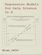Regression Models for Data Science in R (Brian Caffo)

The book gives a rigorous treatment of the elementary concepts of regression models from a practical perspective. The ideal reader for this book will be quantitatively literate and has a basic understanding of statistical concepts and R programming.

•Nonparametric and Semiparametric Models (Wolfgang K. Hardle)

The aim of this book is to present the statistical and mathematical principles of smoothing with a focus on applicable techniques. The necessary mathematical treatment is easily understandable and a wide variety of interactive smoothing examples are given.

•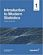Introduction to Modern Statistics (Mine Çetinkaya-Rundel, et al.)

This book puts a heavy emphasis on exploratory data analysis and provides a thorough discussion of simulation-based inference using randomization and bootstrapping, followed by a presentation of the related Central Limit Theorem based approaches.

•Foundations in Statistical Reasoning (Pete Kaslik)

This book is designed for students taking an introductory statistics class. The emphasis throughout the entire book is on how to make decisions with only partial evidence. It focuses on the thought process.

•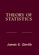Theory of Statistics (James E. Gentle)

This book is directed toward students for whom mathematical statistics is or will become an important part of their lives. It covers classical likelihood, Bayesian, and permutation inference; an introduction to basic asymptotic distribution theory; and modern topics.

•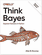O'Reilly® Think Bayes: Bayesian Statistics in Python

If you know how to program, you're ready to tackle Bayesian statistics. With this book, you'll learn how to solve statistical problems with Python code instead of mathematical formulas, using discrete probability distributions rather than continuous mathematics.

•Statistical Inference: Algorithms, Evidence, and Data Science

A masterful guide to how the inferential bases of classical statistics can provide a principled disciplinary frame for the data science of the twenty-first century. Every aspiring data scientist should carefully study this book, use it as a reference.

•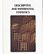Foundations of Descriptive and Inferential Statistics (H. van Elst)

This book aim to provide an accessible though technically solid introduction to the logic of systematical analyses of statistical data to both undergraduate and postgraduate students, in particular in the Social Sciences, Economics, and the Financial Services.

•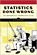Statistics Done Wrong: The Woefully Complete Guide (Reinhart)

Scientific progress depends on good research, and good research needs good statistics. But statistical analysis is tricky to get right, even for the best and brightest of us. You'd be surprised how many scientists are doing it wrong.

•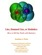Lies, Damned Lies: How to Tell the Truth with Statistics

The goal is to help you learn How to Tell the Truth with Statistics and, therefore, how to tell when others are telling the truth ... or are faking their "news". Covers Data Analysis, Binomial and normal models, Sample statistics, confidence intervals, hypothesis tests, etc.

•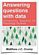Answering Questions with Data : Introductory Statistics

This is a free textbook teaching introductory statistics for undergraduates. Students will learn to select an appropriate data analysis technique, carry out the analysis, and draw appropriate conclusions.

Book Categories
 :All CategoriesTop Free BooksRecent BooksMiscellaneous BooksComputer EngineeringComputer LanguagesComputer ScienceData Science/DatabasesJava and Java EE (J2EE)Linux and UnixMathematicsMicrosoft and .NETMobile ComputingNetworking and CommunicationsSoftware EngineeringSpecial TopicsWeb Programming
Other Categories